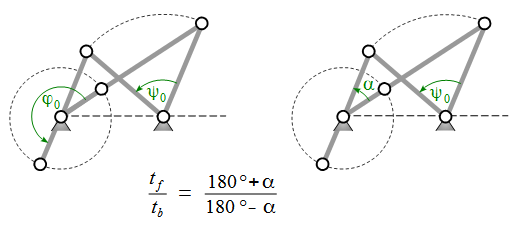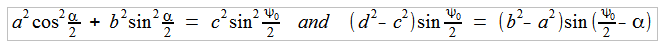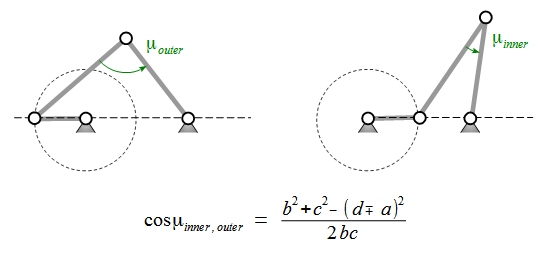Neatly Placed Mail

# npm

## crocker

0.8.6 • Public • Published

# Crank-Rocker -- Design and Analysis.

`Crocker` is a javascript namespace and function library, which has proven successful in designing and analyzing Crank-Rockers as special planar fourbar mechanisms in my lectures. `Crocker.js` is tiny, it weights only 1.5 kB compressed and gzipped.

`Crocker` is not meant to simulate mechanisms. For this and general fourbar mechanisms see fourbar.

Here is a Crank-Rocker animation using the tiny Morphr javascript class and a g2 command queue for vector graphics.

## Geometry

A Crank-Rocker is a specific fourbar mechanism. It is composed of four binary links that are connected to each other by revolute joints. These links are called crank, coupler, rocker and frame. Their lengths are named `a, b, c, d` respectively.The input crank is fully rotatable and performs a complete 360° turn. At the same time the rocker oscillates between its extreme positions `ψ_min` and `ψ_max`. Both crank and rocker are connected to the fixed frame by pivots. The coupler functions as a connecting rod between that two.The rocker's working angular range is named `ψ_0` and corresponds during tracking motion (crank and rocker move unidirectional - counterclockwise here) to the crank's angular range `φ_0`. On their way back they run in opposite directions. The crank's angle is `360° - φ_0` then and the rocker's `-ψ_0`. As a descriptive angle we use the difference `α = 180° - φ_0` here. So if `φ_0` is not 180° or `α` is not 0°, the crank needs different times for the rocker's back and forth motion. This degree of non-uniformity can be expressed by the ratio `t_f/t_b`, which is an important parameter of the engineer's point of view while designing a crank-rocker mechanism and directly relates to `α`. The other important design parameter of course is the rocker's angular range `ψ_0`.

As the four link lengths `a, b, c, d` sufficiently define a specific crank-rocker and `α` and `ψ_0` are added as important design parameters, there must be two relations between those now six parameters. It can be shown that these relations are:Another important value is the transmission angle `μ` between coupler and rocker. Of special interest here is the minimal transmission angle `μ_min` which occurs, when the crank is collinear with the frame. This happens two times while the crank performs a complete rotation.So the minimal transmission angle `μ_min` has to be calculated as `min(μ_inner,μ_outer)`. Please note, that - as a convention - the transmission angle is always considered to be in the range `0 < μ <= 90°`. So in case `μ` happens to be greater than 90°, its supplement angle (`180° - μ`) should be taken instead.

Ideally the transmission angle is always 90°, which isn't possible of course. So a specific design goal with crank-rockers is to maximize the minimal transmission angle. A valuable optimization method regarding this can be applied with `crocker.js`.

See ,  or  for further details.

## Implementation

The `crocker` library consists mostly of small functions. It is no `class`, so you cannot instantiate any Crank-Rocker objects.

The most simple way to use the `crocker` library is with `node.js`.

### Node Example - Simple Crank-Rocker

Here is a table of `Crocker` functions. Please note that these functions are only loosely coupled. Usually the validity of the parameter set handed over to them is checked in the context, from where they are invoked. So please ensure to use valid parameters, as

• `a,b,c,d` are Grashof positive.
• `a` is the smallest link length.
• `ψ_0` is smaller than 180°.
• All angles are provided in radians.

just to name a few.

Function Comment
`.psiMin(a,b,c,d)` Minimal Rocker angle `ψ_min`.
`.psiMax(a,b,c,d)` Maximal Rocker angle `ψ_max`.
`.phiMin(a,b,c,d)` Crank angle `ϕ_min` corresponding to rocker angle `ψ_min`.
`.phiMax(a,b,c,d)` Crank angle `ϕ_max` corresponding to rocker angle `ψ_max`.
`.phi0(a,b,c,d)` Crank angular range `ϕ_0` corresponding to `ψ_0` in unidirectional mode.
`.muInner(a,b,c,d)` Transmission angle `μ_inner` corresponding to crank's inner frame position.
`.muOuter(a,b,c,d)` Transmission angle `μ_outer` corresponding to crank's outer frame position.
`.muMin(a,b,c,d)` Minimal Transmission angle `μ_min`.
`.alfa_tfb(tfb)` Crank angular range difference `α` from time ratio `tf/tb` of rocker's forth and back moving times.
`.a_muMinMax(d,alfa,psi0)` Crank length for maximized minimal transmission angle.

The other functions in the `crocker` library help to determine the missing parameters when four out of the six `a, b, c, d, α, ψ_0` are given. The following table shows those functions with their required arguments:

function `a` `b` `c` `d` `α` `ψ_0`
`.alfa` `*` `*` `*` `*` `-` `-`
`.alfa_abc` `*` `*` `*` `-` `-` `*`
`.alfa_abd` `*` `*` `-` `*` `-` `*`
`.alfa_acd` `*` `-` `*` `*` `-` `*`
`.alfa_bcd` `-` `*` `*` `*` `-` `*`
`.psi0` `*` `*` `*` `*` `-` `-`
`.psi0_abc` `*` `*` `*` `-` `*` `-`
`.psi0_abd` `*` `*` `-` `*` `*` `-`
`.psi0_acd` `*` `-` `*` `*` `*` `-`
`.psi0_bcd` `-` `*` `*` `*` `*` `-`
`.a_bc` `-` `*` `*` `-` `*` `*`
`.a_bd` `-` `*` `-` `*` `*` `*`
`.a_cd` `-` `-` `*` `*` `*` `*`
`.b_ac` `*` `-` `*` `-` `*` `*`
`.b_ad` `*` `-` `-` `*` `*` `*`
`.b_cd` `-` `-` `*` `*` `*` `*`
`.c_ab` `*` `*` `-` `-` `*` `*`
`.c_ad` `*` `-` `-` `*` `*` `*`
`.c_bd` `-` `*` `-` `*` `*` `*`
`.d_ab` `*` `*` `-` `-` `*` `*`
`.d_ac` `*` `-` `*` `-` `*` `*`
`.d_bc` `-` `*` `*` `-` `*` `*`

### Node Example - Optimal Crank-Rocker

Here is a graphics example using `crocker.js` and g2.

## References

 J.M. McCarthy: Geometric Design of Linkages

 J.J. Uicker: Theory of Machines and Mechanisms

## GitCDN

Use the link https://gitcdn.xyz/repo/goessner/crocker/master/crocker.min.js for getting the latest commit as a raw file.

In HTML use ...

`crocker.js` is licensed under the terms of the MIT License. See LICENSE-MIT for details.

## API Reference

See the API Reference for details.

# Change Log

All notable changes to this project will be documented here. This project adheres to Semantic Versioning.

## Keywords

### Install

`npm i crocker`

### Repository

github.com/goessner/crocker